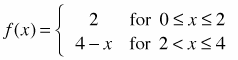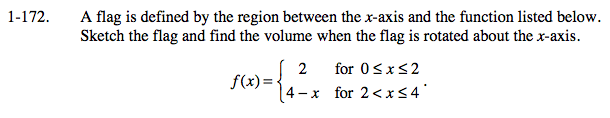### Home > CALC > Chapter 1 > Lesson 1.4.3 > Problem1-172

1-172.
1. A flag is defined by the region between the x-axis and the function listed below. Sketch the flag and find the volume when the flag is rotated about the x-axis. To help you visualize this, use the 1-172 eTool (Desmos). Homework Help ✎Use the eTool below to visualize the flag.
Click the link at right for the full version of the eTool: Calc 1-172 HW eTool

When rotated around the x-axis, it is a cylinder next to a cone, so volume is:

$V=\pi r^{2}h_{1}+\frac{1}{3}\pi r^2h_{2}$